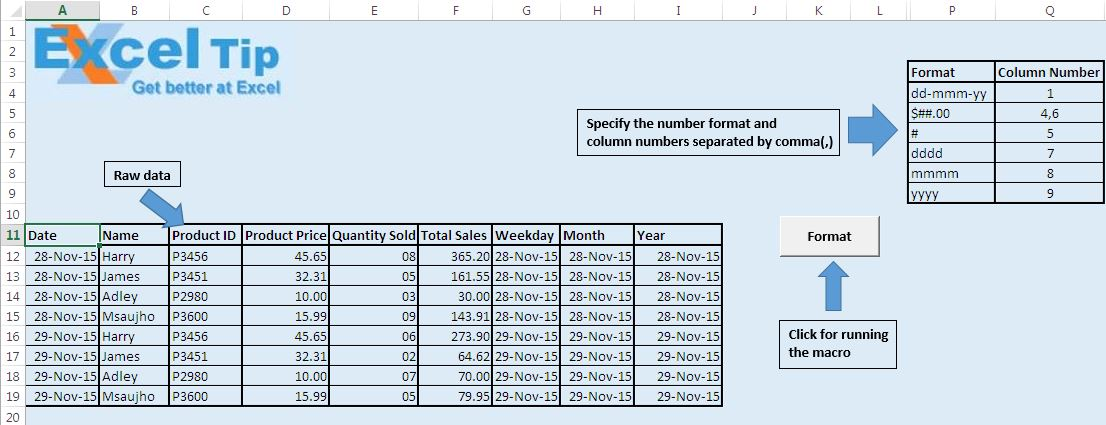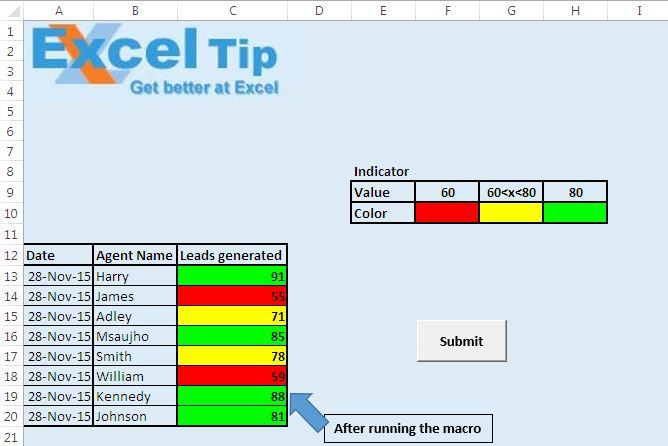# Format data with custom number formats using VBA in Microsoft Excel

In this article, we will create a macro to format the given data in the desired custom number format using VBA.

Raw data for this example consists of sales team data. Raw data contains name, product id, product price, quantity sold and total sales.Before running the macro, one has to specify the custom number format in column P and column number in which one wants to apply the custom number format in the column Q.

Click on the “Format” button to run the “Formatting” macro.Macro will change the formatting of the given data according to the specified custom number format.

Logic explanation

This macro picks the number formatting from the column P and assigns the number formatting to columns specified by column numbers in the column Q.

In this macro, we have use two DO UNTIL Loops for looping. First DO UNTIL Loop is used to loop till all the number formats are applied to columns. The second DO UNTIL Loop is used to find all the different column numbers specified and separated by commas(,).

Code explanation

strCol = Left(txt, InStr(txt, ",") - 1)

Above code is used to separate the column number from the string which contains all the column numbers separated by commas(,).

Columns(CInt(strCol)).NumberFormat = wks.Cells(intRow, 16).Value

Above code is used to apply the custom number format on the specified column.

txt = Right(txt, Len(txt) - InStr(txt, ","))

Above code is used to separate the left out string after the removal of the column number from the defined string.

```Option Explicit

Sub Formatting()

'Declaring variables
Dim wks As Worksheet
Dim intRow As Long
Dim strCol As String
Dim txt As String

'Initializing the variables
Set wks = Worksheets("Format")
intRow = 4

'Looping in 16th column until empty value is found
Do Until IsEmpty(wks.Cells(intRow, 16))

'Assigning the value of custom number format
txt = wks.Cells(intRow, 17)

'Looping and finding all the column number separated by comma(,)
Do Until InStr(txt, ",") = 0

'Getting the column number
strCol = Left(txt, InStr(txt, ",") - 1)
'Assigning the number format
Columns(CInt(strCol)).NumberFormat = wks.Cells(intRow, 16).Value
'Truncating the string for finding the next column number after the comma(,)
txt = Right(txt, Len(txt) - InStr(txt, ","))
Loop

'Assigning the number format
Columns(CInt(txt)).NumberFormat = wks.Cells(intRow, 16).Value
intRow = intRow + 1

Loop

End Sub```